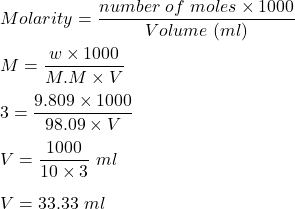What is the volume of 3.00 M sulfuric aid that contain 9.809 g of H2SO4 (98.09g/mol)

Question

What is the volume of 3.00 M sulfuric aid that contain 9.809 g of H2SO4 (98.09g/mol)

in progress 0
5 months 2021-08-18T06:39:17+00:00 1 Answers 7 views 0

1. Given :

Molarity of sulfuric acid solution is 3.0 M.

Amount of sulfuric acid present in solution is 9.809 g.

To Find :

The volume of solution.

Solution :

We know, molarity is given by :Therefore, volume required is 33.33 ml .